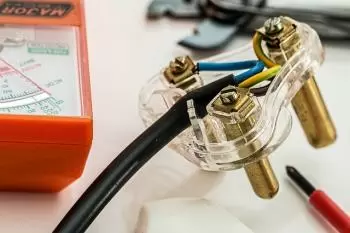# What Is Electric Power? Definition and ConceptElectric power is the proportion of electrical current that is transferred in an electrical circuit per unit of time. That is, the amount of electrical energy that an element generates or dissipates over a period of time.

In the international system of units, the unit of power is expressed in watts (W).

Electric power is generally transferred by electric generators, but it can also be generated by electric batteries and other sources.

The electrical energy absorbed by an electrical device is measured in watt-hours (Wh) or kilowatt-hours (kWh) —1 Wh = 3,600 joules. Electric companies that supply homes and factories generally bill the variable term in kilowatt-hours.

Electric charges flowing through a circuit can transfer some of their energy to obtain mechanical work or heat. Electrical devices convert electrical energy into other forms of energy: heat, motion ( electric motor ), light, etc.

## Electric Power in Direct Current

The electrical power consumed by a resistor in an electrical circuit is the product of the current through a device and the voltage (Joule's law)

P = IR

Where

• I - is the current intensity expressed in amperes at that instant.
• V - is the instantaneous value of the voltage in volts at that instant.
• P - is the power expressed in watts.

## Electric Power in an Alternating Current Circuit

When it comes to sinusoidal alternating current (AC), the average electrical power consumed by a device in an alternating current circuit is a function of:

• the RMS values ​​or root mean square values.

• the voltage between the device terminals.

• of the intensity of current that passes through the electrical device.

Actually, Joule's law can also be applied as we did in the case of a direct current power. In an alternating current, the voltage and current are in "phase." This means that voltage and current have their minimum and maximum values ​​at the same moment.

## What Is the Contracted Power of the Electricity Bill?

The contracted power is the maximum electrical power that, as customers of an electrical company, we can consume at a given time.

To calculate electrical power, the number of electrical appliances that can be used at a supply point and the power they require must be taken into account at the same time.

Generally, the value of the contracted power is expressed in kilowatts (kw).

The contracted power is part of the power term of the electricity bills.

The contracted power must never exceed the maximum allowable power of our electrical installation.

## Examples of Electric Powers

Electrical devices can be generators or consumers of electricity. In any case, all of them have the ability to generate or consume electrical power.

Below we attach examples of the electrical power of some devices. These values ​​can only be taken as a reference since these actual values ​​can vary a lot depending on the model and design.

 Electrical device Electric power Electric scooter 300 - 1000 watts (W) Electric heating 800 - 3000 watts (W) Power of an average home of 90m2 4.6 kilowatts (kW) 0 A photovoltaic panel 250 - 500 watts (W) Kurnool Ultra Mega photovoltaic park, India 1,000 megawatts (MW) Nuclear power station 1,000 - 8,000 (MW)
Author:

Published: September 27, 2021
Last review: September 27, 2021